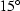Next: Conclusions Up: Numerical Examples Previous: The new R

## Angle results

In the previous section we discussed the possibility of controling the aperture angle in phase-shift migration. Here, we present some synthetic results that show how we can control the angle using the expressions in (15), (16), (12).compan
Figure 11
Angle control; the impulse responses correspond to, (a), (b), (c), and (d) corresponds to the the,,,and the response from the double square root equation (1), respectively.Figure 11 shows the impulse response for the expressions (15), (16), (12). Observe how we limit the aperture angle response with the three different expressions.Next: Conclusions Up: Numerical Examples Previous: The new R
Stanford Exploration Project
4/29/2001